### Parallel Lines Class 9th Mathematics Part Ii MHB Solution

##### Class 9th Mathematics Part Ii MHB Solution

###### Practice Set 2.1
Question 1.

In figure 2.5, line RP||line MS and line DK is their transversal. ∠DHP = 85°

Find the measures of following angles.

i. ∠RHD ii. ∠PHG

Iii ∠HGS iv. ∠MGK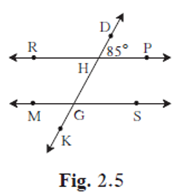Given: RP ∥ line MS and line DK is their transversal.

(i) ∠ DHP + ∠ RHD = 180° (linear pair angle) means that linear pair is a pair of adjacent, supplementary angle. Adjacent means next to each other, and supplementary means that measures of the two angles add up to equal 180 °.

∠ DHP + ∠ RHD = 180°

85° + ∠ RHD = 180° (∠ DHP = 85° given)

∠ RHD = 180° -85°

∠ RHD = 95°

(ii) ∠ RHD ≅ ∠ PHG (vertically opposite angles formed are congruent)

So, ∠ PHG = 95°

(iii) line RP || line MS (given)

∠ DHP ≅ ∠ HGS (corresponding angles) if two parallel line are cut by a transversal, then the pairs of corresponding angle are congruent.

∠ DHP = 85° (given)

So, ∠ HGS = 85°

(iv) ∠ HGS ≅ ∠ MKG (vertically opposite angles formed are congruent)

So, ∠ MKG = 85°

Question 2.

In figure 2.6, line p|| line q and line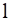and line m are transversals. Measures of some angles are shown. Hence find the measures of ∠ a, ∠ b, ∠ c∠ d.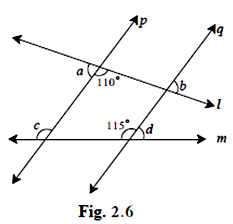Given line P ∥ line Q and line L and M are transversal.

To find: ∠ a, ∠ b, ∠ c∠ d.

Construction: extend G and E in answer diagram.

∠ a + ∠ e = 180° (linear pair angle) means that linear pair is a pair of adjacent, supplementary angle.

Adjacent means next to each other, and supplementary means that measures of the two angles add up to equal 180°.

∠ a + 110° = 180° (given)

∠ a = 180° -110°

∠ a = 70°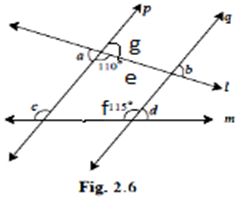∠ a ≅ ∠ g (vertically opposite angles formed are congruent

∠ a = 70° (prove above)

∠ 70° ≅ ∠ g

Line P || line Q and line L transversals (given)

∠ g = ∠ b (corresponding angles)

∠ b = 70°

Line P || line Q and line M is transversal (given)

∠ c ≅ ∠ f (corresponding angles) if two parallel line are cut by a transversal, then the pairs of corresponding angle are congruent.

So, ∠ f = 115° (given)

Then, ∠ c = 115°

∠ d + ∠ f = 180° (linear pair angle)

∠ d + 115° = 180° (given)

∠ d = 180° -115°

∠ d = 65°

Question 3.

In figure 2.7 line /|| line m and line n|| line p. Find ∠ a, ∠ b, ∠ c from the given measure of an angle.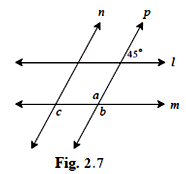Given line L || line M and line P is transversal.

To find: ∠ a, ∠ b, ∠ c

Construction: extend E and D in answer diagram

∠ e ≅ ∠ d (corresponding angle theorem) if two parallel line are cut by a transversal, then the pairs of corresponding angle are congruent.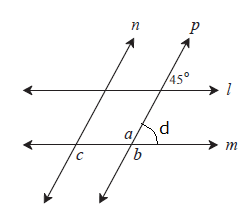� � �

∴ ∠ d = 45° (given above diagram)

∠ a + ∠ d = 180° (linear pair angle)

∠ a + 45° = 180°

∠ a = 180° -45°

∠ a = 135°

∠ a ≅ ∠ b (vertically opposite angles formed are congruent)

∴ ∠ b = 135°

Line N || line P and line M is transversal (given)

∴ ∠ b≅ ∠ c (corresponding angle theorem) if two parallel line are cut by a transversal, then the pairs of corresponding angle are congruent.

∴ ∠ c = 135°.

Question 4.

In figure 2.8, sides of ∠ PQR and ∠XYZ are parallel to each other. prove that, ∠ PQR ≅ ∠ XYZ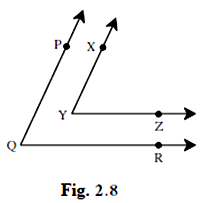Given ∠ PQR AND ∠XYZ are parallel and also YZ and QR are parallel

TO find: ∠ PQR ≅ ∠ XYZ

Construction: extend sag XY such that Q-S-R.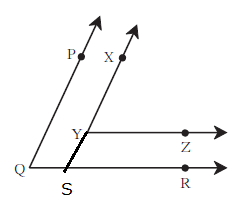PQ|| XY (given)

PQ || XS and QR is transversals (from construction)

∠ PQR ≅ ∠ XSR (corresponding angle theorem) if two parallel line are cut by a transversal, then the pairs of corresponding angle are congruent) ………………………. (1)

YZ||SK and XS is transversals (given)

∠ XYZ ≅ ∠ XSR (corresponding angle theorem) if two parallel line are cut by a transversal, then the pairs of corresponding angle are congruent.) …………………………(2)

Equation (1) and (2) right side is equal

So that, ∠ PQR≅ ∠ XYZ hence proved.

Question 5.

In figure 2.9, line AB || line CD and line PQ is transversal. Measure of one of the angles is given.

Hence find the measures of the following angles.

i. ∠ ART ii. ∠ CTQ

iii. ∠ DTQ iv. ∠ PRB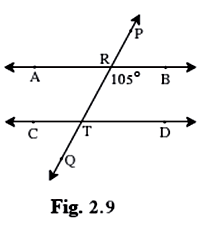Given AB ||line CD and line PQ sis transversal .and∠ PRB 105°and ∠ BRT 105°

To find: ∠ ART, ∠ CTQ, ∠ DTQ, ∠ PRB

∠ ART + ∠ BRT = 180° ° (linear pair angle) means that linear pair is a pair of adjacent, supplementary angle.

Adjacent means next to each other, and supplementary means that measures of the two angles add up to equal 180 °.)

∠ ART + 105° = 180 (∠ BRT = 105 ° given)

∠ ART = 180° -105°

∠ ART = 75°

∠ ART ≅ ∠ PRQ (vertically opposite angles formed are congruent).

So, ∠ PRB = 75° (∵ ∠ ART 75°)

Line AB || line CD line PQ is transversal (given)

∠ BRT ≅ ∠ DTQ (corresponding angle theorem) if two parallel line are cut by a transversal, then the pairs of corresponding angle are congruent).

∠ DTQ = 105 (∠ BRT is 105°)

So that, ∠ DTQ = 105°

∠ ART ≅ ∠ CTQ (corresponding angle theorem) if two parallel line are cut by a transversal, then the pairs of corresponding angle are congruent).

So that, ∠ CTQ = 75° (because ∠ ART is 75°)

###### Practice Set 2.2
Question 1.

In figure 2.18, y = 108°. and x = 71° Are the lines m and n parallel? Justify?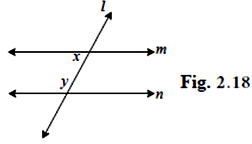Given x = 71°, y = 108°.

To find: Are the lines m and n parallel or not?

X + y = 108° + 71° (already given)

= 179°

X + y ≠ 180°

They from a pair of interior angle which are not supplement.

∴ Line M is not parallel to Line N.

Question 2.

In figure 2.19, if ∠ a≅ ∠ b then prove that line L ∥ line M.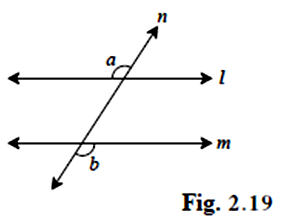∠ a≅ ∠ b

To find: line L ∥ line M

Construction: extend C in figure.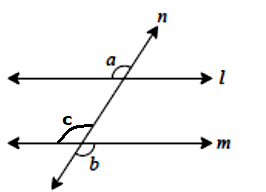∠ a≅ ∠ b (given)

∠ b≅ ∠ c (vertically opposite angle)

∠ a ≅ ∠ c (if whenever an element A is related to an element B and B is related to an element C then A is also related to c that is called transitivity)

But they form a pair of corresponding angle that are congruent.

∴ line L ∥ line M (hence proved).

Question 3.

In figure 2.20, if ∠ a≅ ∠ b and ∠ x≅ ∠ y then prove that line L ∥ line N.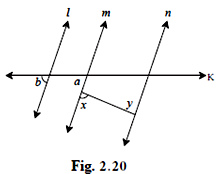Given ∠ a≅ ∠ b and ∠ x≅ ∠ y

To find: line L ∥ line N

∠ a≅ ∠ b (given)

But they form a pair of corresponding angles which are congruent

Line L ∥ line M …………... (1)

∠ x≅ ∠ y (given)

But they form a pair of alternative angle that are congruent

Line M ∥ line N (alternative angle) ………… (2)

Equation (1) and equation (2)

Line L ∥ line N (hence proved).

Question 4.

In figure 2.21, if ray BA || ray DE, ∠ c = 50° and ∠ D = 100°. Find the measure of ∠ ABC.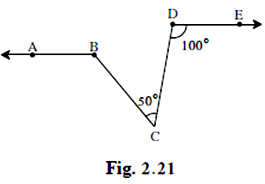(Hint: Draw a line passing through point C and parallel to line AB.).

Given: ray ∠ BA ∥ ∠ DE and ∠ c = 50°, ∠ D = 100°.

To find: ∠ ABC.

Construction: Extend AB such that A-B-F-G.

BA ∥ DE (given)

AG∥ DE(construction) and DC is transversal.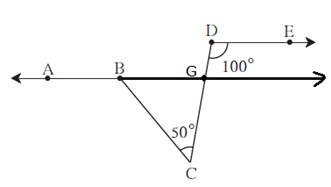∠ D ≅ ∠ GFC ((corresponding angle theorem) if two parallel line are cut by a transversal, then the pairs of corresponding angle are congruent).

∠d = 100° (given)

So that ∠ GFC = 100°

∠ GFC + ∠ BFC = 180(linear pair angle

∠ GFC = 100 (proved above)

∠ GFC + ∠ BFC = 180°

∠ 100 + ∠ BFC = 180°

∴ ∠ BFC = 100-180°

∠ BFC = 80°

In Δ BFC

∠ BFC + ∠ c + ∠ FBC = 180° (sum of angle of Δ)

∠ 80° + 50° + ∠ FBC = 180° (already given value above ∠ BFC and ∠ c)

∠ FBC = 180° -50°

∠ FBC = 130°

∠ ABC + ∠ FBC = 180°

∠ ABC + 50 ° = 180°

∠ ABC = 180-50°

∠ ABC = 130.

Question 5.

In figure 2.22, ray AE || ray BD, ray AF is the bisector of ∠ EAB and ray BC is the bisector of ∠ ABD. Prove that line AF || line BC.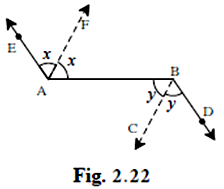Given ray AE∥ ray BD. ray AF is bisector of ∠ EAB and ray BC is the bisector of ∠ Abd.

To find: line AF ∥ line BC

∠ EAB = 2x (ray AF bisector ∠ EAB)

When a line, shape, or angle inti two exactly equal parts is called bisector.

∠ ABD = 2y (ray BC bisector ∠ ABD)

Ray AE ∥ ray BD and Ab is transversal.

∠ EAD ≅ ∠ ABD (alternate angle) two angle formed when a line crosses two other lines, that lie on opposite side of the transversal line and on opposite relative sides of the other lines. If the two lines crossed are parallel, the alternate angles are equal.)

2x = 2y

X = y

∠ FAB∠ ABC

But they form a pair of alternate angle that are congruent.

∴ line AF ∥ line BC (hence proved)

Question 6.

A transversal EF of line AB and line CD intersects the lines at point P and Q respectively. Ray PR and ray QS are parallel and bisectors of ∠ BPQ and ∠ PQC respectively.

Prove that line AB|| line CD.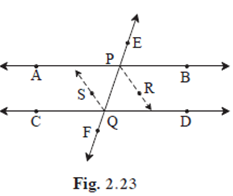ray PR ∥ SQ and PQ is transversal (given)

To find: AB ∥ CD

∠ RPQ ≅ ∠ PQS (alternate angle) two angle formed when a line crosses two other lines, that lie on opposite side of the transversal line and on opposite relative sides of the other lines. If the two lines crossed are parallel, the alternate angles are equal.)

X = y

∠ BPQ = 2x (ray PR bisect ∠ BPQ)

∠ PQC = 2y (ray SQ bisect ∠ PQC)

When a line, shape, or angle inti two exactly equal parts is called bisector.

X = y

2x = 2y (multiply 2 on both side)

∠ BPQ = ∠ PQC

But they form a pair of alternate angle that are congruent.

∴ AB ∥ CD (hence proved)

###### Problem Set 2
Question 1.

Select the correct alternative and fill in the blank in the following statements.

If a transversal intersects two parallel lines then the sum of interior angles on the same side of the transversal is ............
A. 0°

B. 90°

C. 180°

D. 360°

When a transversal intersects two parallel lines, then sum of the interior angles, formed on the same side of the transversal is 180°.

Question 2.

Select the correct alternative and fill in the blank in the following statements.

The number of angles formed by a transversal of two lines is ...........
A. 2

B. 4

C. 8

D. 16

When two parallel lines cut by I third line, the third line is called the transversal .8 angles are formed when parallel lines M and N are cut by a transversal line T.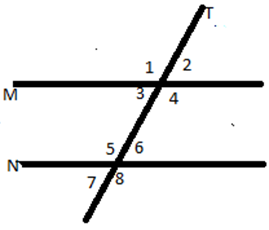Question 3.

Select the correct alternative and fill in the blank in the following statements.

A transversal intersects two parallel lines. If the measure of one of the angles is 40° then the measure of its corresponding angle is .............
A. 40°

B. 140°

C. 50°

D. 180°

A transversal intersects two parallel line so; corresponding angle is equal so that corresponding angle is also 40°

Question 4.

Select the correct alternative and fill in the blank in the following statements.

In ΔABC∠ A =760, ∠ B = 480, ∠ C = …
A. 66°

B. 56°

C. 124°

D. 28°

∠ A + ∠ b + ∠ C = 180° (the sum of the measures of the interior angle of a triangle is 180°

∠ A + ∠ B + ∠ C = 180°

∠ 76 + ∠ 48 + ∠ C = 180°

∠ 124 + ∠ c = 180°

∠ C = 180-124

∠ C = 56°

Question 5.

Select the correct alternative and fill in the blank in the following statements.

Two parallel lines are intersected by a transversal. If measure of one of the alternate interior angles is 75° then the measure of the other angle is .............
A. 105°

B. 15°

C. 75°

D. 45°

If measure of one of the alternate interior angles is 75° then the measure of the other angle is 75° because two parallel are intersected by transversal.

Question 6.

Ray PQ and ray PR are perpendicular to each other. Points B and A are in the interior and exterior of ∠QPR respectively. Ray PB and ray PA are perpendicular to each other.

Draw a figure showing all these rays and write -

i. A pair of complementary angles

ii. A pair of supplementary angles.

iii. A pair of congruent angles.

iii. A pair of congruent angles.

The figure is attached below: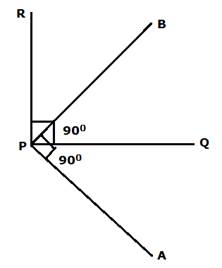(i) ∠ RPB + ∠ BPQ = 90°

(ii) ∠ RPQ + ∠ BPA = 180°

(iii)∠ RPQ = ∠ BPA (congruent angle)

(iv) ∠ RPB = ∠ QPA (congruent angle)

Question 7.

Prove that, if a line is perpendicular to one of the two parallel lines, then it is perpendicular to the other line

The diagram is given below: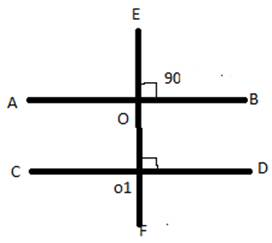To find: transversal line will be perpendicular to other parallel line.

AB ∥ CD and EF is transversal both.

So, ∠ EOB = ∠ O O1D = 90°

So that transversal line will perpendicular to other parallel line also. (hence proved)

Question 8.

In figure 2.24, measures of some angles are shown. Using the measures find the measures of ∠ x and ∠ y hence show that line l ∥ line m.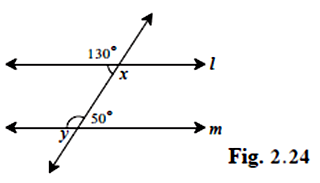Given: value of ∠ x and ∠ y

To find: line l ∥ line m

∠ y = 180-50 ° (linear pair angle) means that linear pair is a pair of adjacent, supplementary angle. Adjacent means next to each other, and supplementary means that measures of the two angles add up to equal 180 °.)

∠ y = 130 °

Transversal line making same angle to both line.

So, that line L ∥ line M.

Question 9.

Line AB ∥ CD ∥ Line EF and line QP is their transversal. If Y: z = 3:7 then find the measure of ∠x. (See figure 2.25.)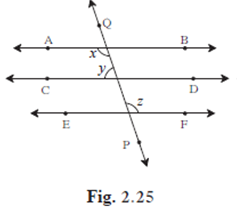Given line AB ∥ CD ∥ Line EF and line QP is their transversal.

To find: ∠ X.

AB ∥ CD ∥ EF (linear pair angle) (linear pair angle) means that linear pair is a pair of adjacent, supplementary angle .Adjacent means next to each other ,and supplementary means that measures of the two angles add up to equal 180 °.

X = z (alternate interior angles) …………. (1)

Alternate interior angles are a pair of angles on the inner side of each of those two lines but on opposite side of the transversal.

Y: z = 3:7 (given)

Let the common ratio between y and z be a

X + y = 180° (co -interior angles on the same side of the transversal)

Z + y = 180° (using equation 1)

7a + 3a = 180°

10a = 180°

A = 18°

∴ x = 7a

X = 7× 18°

X = 126°

Question 10.

In figure 2.26, if line Q ∥ line R and p is their transversal and if a = 80° find the values of f and g.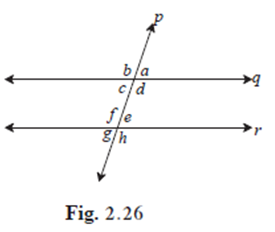Given line Q ∥ line R and p is their transversal. and a = 80°

To find: values of F and G.

∠ a = 80°

∠ a = ∠ c = 80° (vertically opposite angle)

∠ c + ∠ f = 180° (angle made on the same side of parallel lines are supplementary means their sum is 180°)

∠ 80° + ∠ f = 180°

∠ f = 180 = 80

∠ f = 100 °

∠ f + ∠ g = 180° ° (linear pair angle) means that linear pair is a pair of adjacent, supplementary angle. Adjacent means next to each other, and supplementary means that measures of the two angles add up to equal 180.)

∠ 100 + ∠ g = 180

∠ g = 180-100

∠ g = 80°

Question 11.

In figure 2.27, if line AB || line CF and line BC || line ED then prove that ∠ ABC = ∠ FDE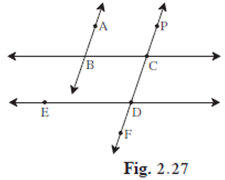Given: Line AB ∥ CF and line BC ∥ ED

To find: ∠ ABC = ∠ FDE

Construction: G and h in diagram.

AB ∥ CD and BC is transversal both

So, ∠ ABC = ∠ PCG (linear pair angle)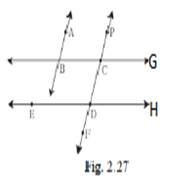BC∥ ED and PF is transversal line to both

So, ∠ ECG = ∠ CDH

∠ CD = ∠ FDE (Both angle is opposite)

∴ ∠ ABC = ∠ FDE (hence proved)

Question 12.

In figure 2.28, line PS is a transversal of parallel line AB and line CD. If Ray QX, ray QY, ray RX, ray RY are angle bisectors, then prove that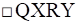is a rectangle.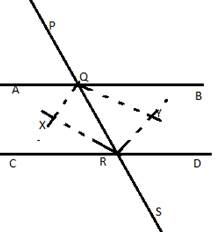Given: PS is transversal of parallel line AB and line CD.

To find: QXRY is rectangle.

∠ AQR + ∠ CRQ = 180°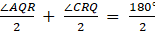(divide by 2)

∠ XQR + ∠ XRQ = 90°

[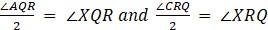QX and RX are bisector)

In Δ XQR

∠ XQR + ∠ XRQ + ∠ QXR = 180°

90° + ∠ QXR = 180° (∠ XQR + ∠ XRQ = 180° proved above)

∠ QXR = 180° -90°

∠ QXR = 90°

Similarly, ∠ QYR = 90°

∠ AQR + ∠ BQR = 180 (straight line)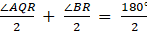(divide by 2)

∠ XQR + ∠ YQR = 90° (QX and QY are bisector ∠)

∠ XQY = 90°

Similarly, ∠ XRY = 90°

If any quadrilateral has all the angle 90° it is a rectangle, so that QXRY is rectangle.

## PDF FILE TO YOUR EMAIL IMMEDIATELY PURCHASE NOTES & PAPER SOLUTION. @ Rs. 50/- each (GST extra)

SUBJECTS

HINDI ENTIRE PAPER SOLUTION

MARATHI PAPER SOLUTION
SSC MATHS I PAPER SOLUTION
SSC MATHS II PAPER SOLUTION
SSC SCIENCE I PAPER SOLUTION
SSC SCIENCE II PAPER SOLUTION
SSC ENGLISH PAPER SOLUTION
SSC & HSC ENGLISH WRITING SKILL
HSC ACCOUNTS NOTES
HSC OCM NOTES
HSC ECONOMICS NOTES
HSC SECRETARIAL PRACTICE NOTES

2019 Board Paper Solution

HSC ENGLISH SET A 2019 21st February, 2019

HSC ENGLISH SET B 2019 21st February, 2019

HSC ENGLISH SET C 2019 21st February, 2019

HSC ENGLISH SET D 2019 21st February, 2019

SECRETARIAL PRACTICE (S.P) 2019 25th February, 2019

HSC XII PHYSICS 2019 25th February, 2019

CHEMISTRY XII HSC SOLUTION 27th, February, 2019

OCM PAPER SOLUTION 2019 27th, February, 2019

HSC MATHS PAPER SOLUTION COMMERCE, 2nd March, 2019

HSC MATHS PAPER SOLUTION SCIENCE 2nd, March, 2019

SSC ENGLISH STD 10 5TH MARCH, 2019.

HSC XII ACCOUNTS 2019 6th March, 2019

HSC XII BIOLOGY 2019 6TH March, 2019

HSC XII ECONOMICS 9Th March 2019

SSC Maths I March 2019 Solution 10th Standard11th, March, 2019

SSC MATHS II MARCH 2019 SOLUTION 10TH STD.13th March, 2019

SSC SCIENCE I MARCH 2019 SOLUTION 10TH STD. 15th March, 2019.

SSC SCIENCE II MARCH 2019 SOLUTION 10TH STD. 18th March, 2019.

SSC SOCIAL SCIENCE I MARCH 2019 SOLUTION20th March, 2019

SSC SOCIAL SCIENCE II MARCH 2019 SOLUTION, 22nd March, 2019

XII CBSE - BOARD - MARCH - 2019 ENGLISH - QP + SOLUTIONS, 2nd March, 2019

HSC Maharashtra Board Papers 2020

(Std 12th English Medium)

HSC ECONOMICS MARCH 2020

HSC OCM MARCH 2020

HSC ACCOUNTS MARCH 2020

HSC S.P. MARCH 2020

HSC ENGLISH MARCH 2020

HSC HINDI MARCH 2020

HSC MARATHI MARCH 2020

HSC MATHS MARCH 2020

SSC Maharashtra Board Papers 2020

(Std 10th English Medium)

English MARCH 2020

HindI MARCH 2020

Hindi (Composite) MARCH 2020

Marathi MARCH 2020

Mathematics (Paper 1) MARCH 2020

Mathematics (Paper 2) MARCH 2020

Sanskrit MARCH 2020

Important-formula

THANKS